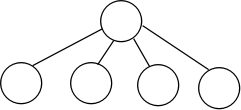## 数据结构——并查集 转

o
osc_1ee7cxmx

### 二、并查集的基本操作及实现

#### 1.初始化

``````int father[maxn];//父节点的索引

void init(int N)//从1开始初始化N个结点
{
for(int i = 1;i <= N;i++)
{
father[i] = i;
}
}``````

#### 2.查询

``````int find(int x)//查询编号为x的结点的根结点
{
if(father[x] == x)
return x;
else
return find(father[x]);
}````````````int find(int x)//查询编号为x的结点的根结点
{
if(father[x] == x)
return x;
else
return father[x] = find(father[x]);//路径压缩
//return find(father[x]);
}``````

#### 3.合并

``````void unite(int x, int y)//合并x和y所在的集合
{
x = find(x);
y = find(y);
if(x == y)//如果x和y已在同一集合，那就打扰了
return;
father[x] = y;
}``````

o

### osc_1ee7cxmx#### 暂无文章

IamOkay
30分钟前
31
0

40分钟前
6
0

① 进程与线程 程序、进程、线程、协程的概念 程序： 用某种语言编写的一组指令的集合，即指一段静态的代码； 进程：简单地说就是一个正在执行的程序或应用，是资源分配的最小单位； 线程：线...

Arno_pei
53分钟前
0
0
08VulKan——描述符布局、缓冲、描述符池和描述符集

7
0

chipo

18
0